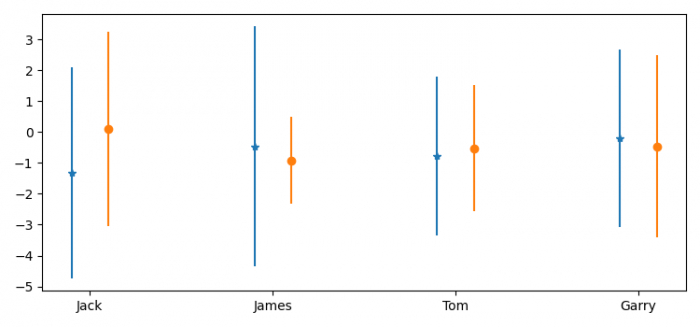# How to avoid overlapping error bars in matplotlib?

To avoid overlapping error bars in matplotlib, we can take the following steps −

## Steps

• Set the figure size and adjust the padding between and around the subplots.

• Create a list of names.

• Get the data points for y1 and y2, and errors ye1, ye2.

• Create a figure and a set of subplots.

• Create a mutable 2D affine transformation, trans1 and trans2.

• Plot y versus x as lines and/or markers with attached errorbars.

• To display the figure, use show() method.

## Example

import numpy as np
import matplotlib.pyplot as plt
from matplotlib.transforms import Affine2D

plt.rcParams["figure.figsize"] = [7.50, 3.50]
plt.rcParams["figure.autolayout"] = True

x = ['Jack', 'James', 'Tom', 'Garry']

y1, y2 = np.random.randn(2, len(x))

ye1, ye2 = np.random.rand(2, len(x))*4+0.3

fig, ax = plt.subplots()

trans1 = Affine2D().translate(-0.1, 0.0) + ax.transData
trans2 = Affine2D().translate(0.1, 0.0) + ax.transData

er1 = ax.errorbar(x, y1, yerr=ye1, marker="*", linestyle="none", transform=trans1)
er2 = ax.errorbar(x, y2, yerr=ye2, marker="o", linestyle="none", transform=trans2)

plt.show()

## Output

It will produce the following output −